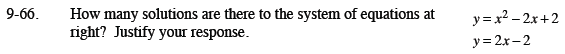Home > CCA2 > Chapter 9 > Lesson 9.2.2 > Problem9-66

9-66.
1. How many solutions are there to the system of equations at right? Justify your response. Homework Help ✎

2. y = x2 − 2x + 2
y = 2x − 2The first equation is a parabola, the second equation is a line.
How many times can a line and parabola intersect?

Graph the equations or solve the system algebraically to determine the number of solutions.

There is only one point of intersection. What is it?